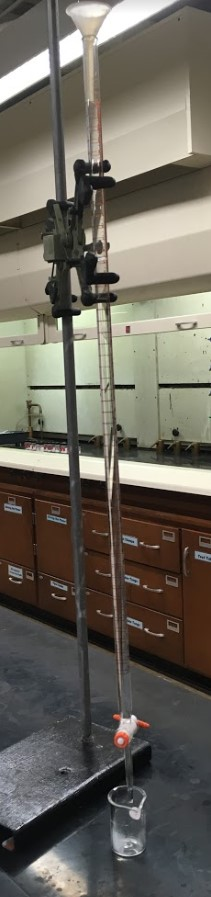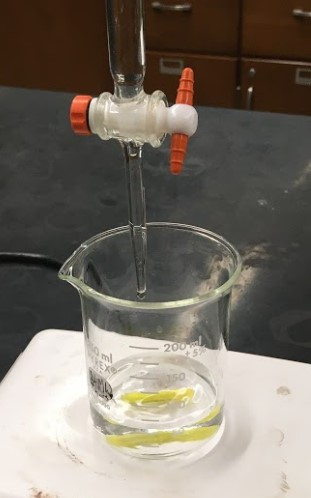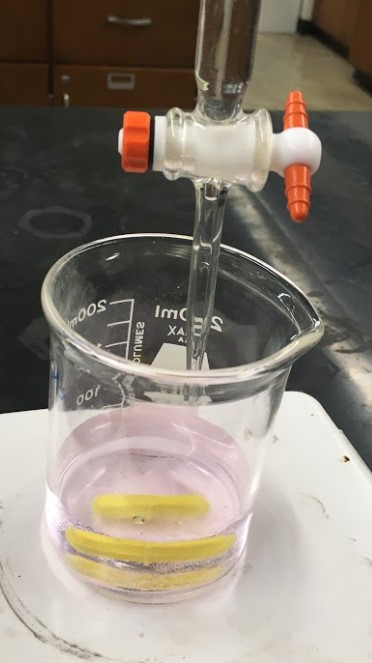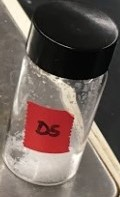# 6: Titration of an Unknown Acid

$$\newcommand{\vecs}{\overset { \rightharpoonup} {\mathbf{#1}} }$$ $$\newcommand{\vecd}{\overset{-\!-\!\rightharpoonup}{\vphantom{a}\smash {#1}}}$$$$\newcommand{\id}{\mathrm{id}}$$ $$\newcommand{\Span}{\mathrm{span}}$$ $$\newcommand{\kernel}{\mathrm{null}\,}$$ $$\newcommand{\range}{\mathrm{range}\,}$$ $$\newcommand{\RealPart}{\mathrm{Re}}$$ $$\newcommand{\ImaginaryPart}{\mathrm{Im}}$$ $$\newcommand{\Argument}{\mathrm{Arg}}$$ $$\newcommand{\norm}{\| #1 \|}$$ $$\newcommand{\inner}{\langle #1, #2 \rangle}$$ $$\newcommand{\Span}{\mathrm{span}}$$ $$\newcommand{\id}{\mathrm{id}}$$ $$\newcommand{\Span}{\mathrm{span}}$$ $$\newcommand{\kernel}{\mathrm{null}\,}$$ $$\newcommand{\range}{\mathrm{range}\,}$$ $$\newcommand{\RealPart}{\mathrm{Re}}$$ $$\newcommand{\ImaginaryPart}{\mathrm{Im}}$$ $$\newcommand{\Argument}{\mathrm{Arg}}$$ $$\newcommand{\norm}{\| #1 \|}$$ $$\newcommand{\inner}{\langle #1, #2 \rangle}$$ $$\newcommand{\Span}{\mathrm{span}}$$$$\newcommand{\AA}{\unicode[.8,0]{x212B}}$$

Objectives

• To perform an analytical titration.
• To standardize a basic solution.
• To determine the molecular mass of an unknown acid.

Chemical reactions between acids and bases are important processes. These reactions can be used to synthesize new substances or to analyze the quantity of a pure substance or of a compound in a mixture. In this experiment, you will first determine the concentration of a base, NaOH, and then use this standardized base to determine the molar mass of an unknown weak acid. Based on the molar mass you will determine the identity of the unknown weak acid.

In water strong acids produce hydronium ions and strong bases produce hydroxide ions. The reaction of a strong acid with a strong base is represented with the chemical reaction shown in Equation 1. Chemists regard this as a reaction that goes to completion; that is all of the reactants are converted to products.

$\ce{H_{3}O^{+}} + \ce{OH^{-}} \rightarrow 2\ce{H_{2}^O} \label{1}$

The reaction between weak acids and strong bases is represented by Equation 2.

$\ce{HA} + \ce{OH^{-}} \rightarrow \ce{H_{2}O} + \ce{A^{-}} \label{2}$

This reaction also goes to completion. In this experiment you will use the reaction in Equation 3 to determine the molar concentration of the strong base NaOH using the weak, monoprotic acid potassium hydrogen phthalate (KHP), where P- is the phthalate group.

$\ce{HP^{-}} + \ce{OH^{-}} \rightarrow \ce{P^{2-}} +\ce{H_{2}O} \label{3}$

The method of analysis will be a titration of acid with base. In the first part of the experiment, you will standardize (determine the exact concentration of) your sodium hydroxide solution. We will determine the concentration of the solution by titrating a known mass of a known acid with your sodium hydroxide solution, using an acid-base indicator to find the endpoint of the titration. In the second part of the experiment, we will determine the identity of an acid by titrating a known mass with out standardized NaOH solution and determining its molecular weight.

## Procedure

Safety and Waste Disposal

• The solutions can be disposed of down the drain.

### Part A: Standardization of a Sodium Hydroxide Solution

In this part of the experiment you will prepare and standardize a sodium hydroxide solution.

###### Step 1From the carboy obtain about 400 mL of the NaOH solution into your 1L plastic bottle. Label the container appropriately. You will determine the more precise value of the molarity of the NaOH solution to 3 significant figures. The remainder of the base that you do not use this week will be kept in your cupboards for next week. Do NOT dispose of any remaining base at the end of the lab period.

###### Step 2Weigh ~ 0.5 g of KHP into a 250 mL beaker and record the weight exactly.

###### Step 3Add about 50 mL of deionized water to dissolve the KHP. It is not important that this volume be exact.

###### Step 4Add 4 drops of phenolphthalein

###### Step 5Fill a clean, dry 50-mL buret with the NaOH solution. Allow some solution to run out the tip to remove the air bubbles.

###### Step 6

Record the initial volume to two decimal places. (It need not be exactly 0.00.)

###### Step 7Place the flask containing your KHP solution on the stir plate with a stir bar in the solution. Turn on the stir plate to a low but steady pace.

###### Step 8You will now add NaOH from the buret to the beaker. You are looking for the point where the solution turns from colorless to a slight pink. This is the endpoint.

###### Step 9

Record the final volume of NaOH in your buret to two decimal places. The difference between the final measurement and the initial is the volume of NaOH used.

###### Step 10

Determine the concentration of NaOH as shown in the Calculations section.

###### Step 11

Calculate the molarity of the NaOH solution to 4 significant figures.

###### Step 12

Repeat the titration with fresh samples of KHP until you have two concentrations that agree within 1.5 %.

### Part B: Determining the Molecular Mass of an Unknown Acid

In this part of the experiment you will use your standardized sodium hydroxide solution to titrate an unknown acid and determine its molecular mass.

###### Step 1Obtain your unidentified acid. Be sure to record the unknown code in a prominent place in your notebook.

###### Step 2In a 250-mL beaker, weigh out 0.3 to 0.4 g of your unidentified acid and record the mass exactly.

###### Step 3Dissolve the acid in approximately 50 mL of deionized water.

###### Step 4Add about 4 drops of indicator.

###### Step 5Place the beaker on a magnetic stir plate and add a stir bar.

###### Step 6Titrate the acid with your standardized base, recording the amount of base necessary to exactly reach the endpoint.

###### Step 7

Calculate the molecular weight of the unknown acid. (see Calculations).

###### Step 8

Repeat the determination.

###### Step 9

Weigh out two more samples of your unknown, increasing or decreasing the mass to have the volume of NaOH used be around 25mL.

###### Step 10

Titrate these samples and record your results and any observations.

###### Step 11

Calculate the molecular weight of the acid. Your results should agree to within 2%.

###### Step 12

Determine the identity of your unknown based on your average molecular mass.

### Part A – Standardization of a Sodium Hydroxide Solution

Data Trial 1 Trial 2 Trial 3 (Trial 4)*
Mass of $$\ce{KHP}$$
Initial $$\ce{NaOH}$$ buret reading:
Final $$\ce{NaOH}$$ buret reading:
Volume of $$\ce{NaOH}$$ used:

###### Step 1

Calculate the moles of $$\ce{KHP}$$.

###### Step 2

Calculate the volume of $$\ce{NaOH}$$.

###### Step 3

Calculate the molarity of the $$\ce{NaOH}$$ solution to 4 significant figures.

###### Step 4

Determine if your result of the standardization value for $$\ce{NaOH}$$ is after two titrations, is within the tolerance. Calculate the percent difference of two  titrations.

$\%\: difference = \frac{|M_{1} - M_{2}|}{M_{2}} \times 100 \label{4}$

Calculations Trial 1Trial 1Trial 1Trial 1 Trial 2 Trial 3 (Trial 4)*
Moles of $$\ce{KHP}$$ used:
Moles of $$\ce{NaOH}$$ titrated:
Molarity of $$\ce{NaOH}$$:

*If necessary

• Average molarity of $$\ce{NaOH}$$ solution: ___________________ M

In the space below, clearly show all calculations for your Trial 1 data only:

### Part B – Determining the Molecular Mass of an Unknown Acid

Unknown Number of Solid Acid: ________________

Data Trial 1 Trial 2 Trial 3 (Trial 4)*
Mass of acid used:
Initial $$\ce{NaOH}$$ buret reading:
Final $$\ce{NaOH}$$ buret reading:
Volume of $$\ce{NaOH}$$ used:

###### Step 1

Calculate the moles of base used to reach the endpoint

###### Step 2

These are monoprotic weak acids so the moles of base equal the moles of acid present at the equivalence point.

###### Step 3

Using the mass of the unidentified acid you measured and the moles of acid you calculated, determine the MW of the unidentified acid.

$MW=\frac{grams\: in\: sample}{moles\: in\: sample} = \frac{g}{mol} \label{5}$

Calculations Trial 1 Trial 2 Trial 3 (Trial 4)*
Moles of $$\ce{NaOH}$$ used:
Moles of $$\ce{H^{+}}$$ titrated:
Molecular Mass

*Only three trials are required, but space for a fourth is given if needed.

Average equivalent mass of unknown acid: ___________________ $$g·eq^{–1}$$

In the space below, clearly show all calculations for your Trial 1 data only:

Unknown Mass Identification:

## Unknown Possibilities:

Acid MW (g/mol)
Crotonic 86.09
Mandelic 152.14
Potassium hydrogen phthalate 204.23
Propionic 74.08
Benzoic 122.12
Sodium hydrogen sulfite 104.06

6: Titration of an Unknown Acid is shared under a not declared license and was authored, remixed, and/or curated by LibreTexts.